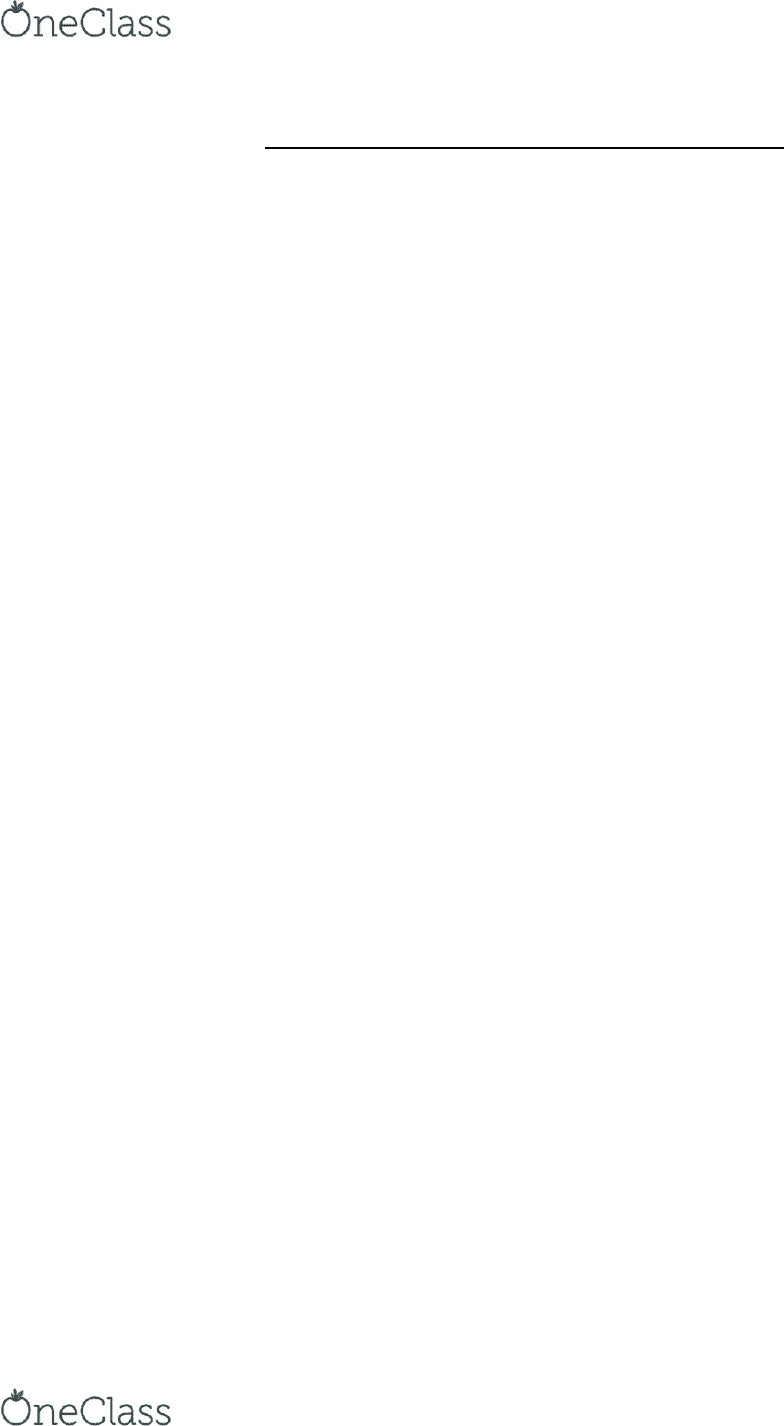Textbook Notes (280,000)
US (110,000)
UNF (100)
ECO (20)
ECO2023 (10)
Chapter 6

# ECO2023 Chapter Notes - Chapter 6: Normal Good, Demand Curve, Inferior Good

Department
Economics
Course Code
ECO2023
Professor
John Slattery
Chapter
6

This preview shows half of the first page. to view the full 3 pages of the document.Chapter 6 Elasticity: The Responsiveness of Demand and Supply
Elasticity is used to measure how one economic variable responds to changes in another
economic variable.
o Knowing price elasticity of demand allows you to compute the effect of a price change
on the quantity demanded
o Price elasticity of supply The responsiveness of quantity supplied of a good to change
in its price
6.1 The Price Elasticity of Demand and Its Measurement
o Price of elasticity of demand- the measure of the responsiveness of the quantity
demanded to a change in price.
o Measuring the Price Elasticity of Demand
Using the slope of the demand curve, telling how much quantity changes as
price changes
Value computed for slope can change dramatically, depending on units used for
quantity and price.
use percentage changes
Price elasticity of demand = (Percentage in quantity demanded)/
(Percentage change in price)
Price elasticity of demand is not the same as the slope of the demand
curve
Price elasticity of demand is always negative
o -3 is larger than -2 in economics
o Elastic Demand and Inelastic Demand
Elastic Demand when the percentage change in quantity demand is greater
than percentage change in price, so the price elasticity is greater than 1 in
absolute value
Inelastic Demand Demand is inelastic when the percentage change in quantity
demanded is less than the percentage change in price, so the price is less than 1
in absolute value.
Unit-elastic Demand when percentage change in quantity demanded is equal
to the percentage change in price so the price elasticity is equal to 1 in absolute
value
o The Midpoint Formula
See Midpoint Formula
o Polar Cases of Perfectly Elastic and Perfectly Inelastic Demand
Perfectly inelastic demand when demand curve is a vertical line, where the
quantity demanded is completely unresponsive to price and the price elasticity
of demand equals zero
Perfectly elastic demand when demand curve is a horizontal line, the quantity
demanded is infinitely responsive to price and the price elasticity of demands
equals infinity
find more resources at oneclass.com
find more resources at oneclass.com